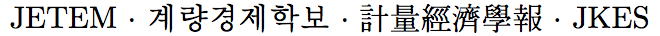Journal of Economic Theory and Econometrics: Journal of the Korean Econometric Society
Home

About this journal

Submit your article

Archive

Search

Journal of Economic Theory and EconometricsJournal of the Korean Econometric Society

• #### Minki Hong   (Korea Labor Institute)Abstract

This paper derives a multinomial logit form for dynamic discrete choice models of optimal stopping and suggests a two-step estimator. The first stage estimates nonparametrically the choice probability of terminal state next period. The second stage is an implementation of modified multinomial logit estimation. This paper also suggests semiparametric conditional choice probability (CCP) estimators for discrete choice dynamic models allowing for unobserved individual heterogeneity. I describe a modified Expectation-Maximization (EM) algorithm that involves nonparametric first stage estimation.

Keywords
Dynamic Model, Optimal Stopping, EM algorithm

JEL classification codes
C14, C25
Links

KCI list
Korean ES
JETEM at SCOPUS

Powered by MathJax